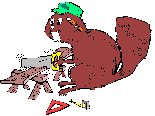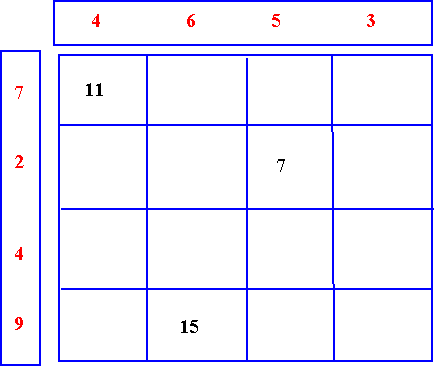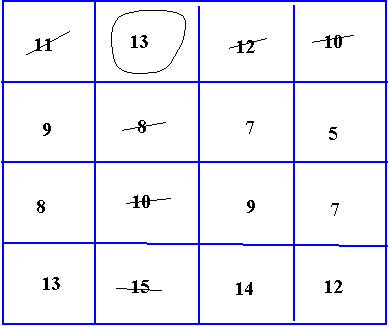# Making Maths: Be a Mathemagician

##### Age 7 to 11Challenge LevelHave you noticed how often magic squares pop up in mathematics? They can be different sizes (a three by three square or even a five by five square), use different numbers (single or more digits) and have different totals (15, 34 or something completely different). There are many variations on how magic squares work.

Here is one type of magic square that you can make to baffle and bewilder friends? Is there a trick to making them? Not a trick but certainly a method.

This example uses a four by four square, but you can draw whatever size square you choose.

You will need :
Paper and a pencil.
A ruler.
Scissors.

• On the outside of the square, write a series of numbers.
• Add the numbers together to find their total. Write the total on a card.
• The total of the numbers below is 40.
• You might want to adjust your numbers to get a particular total.• Add the number at the beginning of a row with the number above the column and write the total in the corresponding cell on the matrix.
• E.g. Above, 7 has been added to 4 and the sum, 11, placed in the corresponding cell. 2 has been added to 5 and the sum, 7, placed in the corresponding cell.
• Continue until the matrix is completed and then cut it out.• Find a friend to baffle .
• Put the card with the total on in an envelope and hand it to your friend.
• Ask your friend to choose any number from the top row and to circle it.
• Then, cross out all of the other numbers in that row, and the numbers in the column the number is in.
• In the second row, circle a number that is not crossed out.
• Cross out the remaining numbers in its row and column.
• Do the same in the other two rows.